﻿ CV [Constant-Velocity] Inverse Crank [Special]

# CV [Constant-Velocity] Inverse Crank

### Constant-Velocity Inverse Crank

CV [Constant Velocity] Inverse Crank is similar to the Y–Inverse Sinusoid Motion-Law.

We urge you to use this motion-law in preference to the Y-Inverse Sinusoid.

This segment can be used more than once in one machine cycle, whereas the Y-Inverse-Sinusoid can be used only one time.

This segment is best explained by considering the X and Y motion components of a Point that is at the end of a rotating Part or Crank.

When the Crank rotates with constant angular velocity, the horizontal* motion of a Point at the end of the Crank is 'Simple-Harmonic-Motion' [ a Sine function]

When you apply the 'Constant-Velocity Inverse-Crank' segment to a Crank, the Point can be made to move with a constant linear horizontal* velocity for a portion of the crank's rotation. Thus, the crank can make a full rotation in each machine cycle, but it does not rotate at constant velocity.

* Actually, the motion of a Point on a Crank projected on to any line is Simple-Harmonic-Motion.

#### SEGMENT-EDITOR

In the Segment Editor - see image below with Segment-Parameters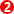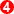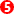You can set the:

 • Minimum Velocity: or the Angle, relative to the Base-Value of the Minimum-Velocity
 • Rel Start: the angle relative to• Rel End: the angle relative toIf you use the Flexible-Polynomial Segment before and after this segment, you can:

 • Use the Blend Control Button to make the End of the Flexible-Polynomial Segment match the start of this Segment.
 • Use the Blend Control Button to make the Start of the Flexible-Polynomial Segment match the end of this Segment.Segment-Parameters of the 'Constant-Velocity InverseSchematic of Crank (green) made to give a constant linear velocity between the Start Angle(4) and End Angle(5).
You can edit the angles 2, 4 and 5 in the Segment Editor.

#### Segment Editor : Segment-Parameters

Note: These angles are on the Y-axis of the motion. They are NOT angles on the X-axis.

Minimum Velocity: the Angle of Minimum Velocity

The angle, after the Base-Value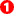* of the Crank, at which the angular velocity of the Crank is a minimum. At all other angles, within the range of this segment, the angular velocity of the Crank is greater than the Minimum Velocity. In full, we call this parameter the Angle of Minimum Velocity.

The direction of the Constant-Velocity vector for Point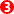on the Crank is projected onto an imaginary Line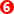, which is perpendicular to the Angle of Minimum Velocity.

*The Base-Valueis the parameter defined in the Motion-Dimension FB dialog for the Crank, not by the position of the end of the Previous-Segment.

Rel. Start: the Relative Angle before the Minimum Velocity Angle

The angle, before from the Angle of Minimum Velocity, at which the Constant-Velocity of Pointstarts.

Rel. End: the Relative Angle after the Minimum Velocity Angle

The angle, after the Angle of Minimum Velocity, at which the Constant-Velocity of Pointends.

Note:

Rel. Start is less than Min Vel, and Rel. End more than Min Vel, when the Crank rotates Counter-Clockwise.

Rel. Start is more than Min Vel, and Rel. End less than Min Vel, when the Crank rotates Clockwise.

The velocity of the Pointwhen projected on to the Lineis constant while the crank moves within the angular range of:

Start Angle =+-...to...

End Angle =++The linear velocity and the length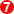of the Constant-Velocity of the Point along the line depends on the:

 • Segment-Width
 • Length of the Rocker/Crank
 • Segment-Parameters Rel Start Angle + Rel End Angle.Two CV Inverse-Velocity Segments in one Motion

An example motion with four segments and two CV Inverse Crank

Segment 1:

 • Is the 'Constant-Crank-Velocity, from 0 to 73º along the X–axis
 • The Constant-Crank-Velocity Parameters are:
 o Angle of Minimum Velocity = 0º
 o Relative Start Angle = -30º
 o Relative End = 30º

With these Parameters:

 o The Y–axis Value is -30º, when the Master Machine Angle (MMA) is 0º
 o The Y–axis Value is 30º when the Master Machine Angle is at 73º

The Velocity, Acceleration and Jerk at the end of the Previous-Segment are made to match Start of this Segment.

The Position at the end of the Previous-Segment (the end of the Motion) is usually = 'Position at Start + 360º'

This makes sure the Crank rotates one time for each for each Machine Cycle

Segment 2:

 • Is a 'Flexible-Polynomial' Motion-Law, from 73 to 180º along the X–axis
 • All of the motion derivatives are made to Match the end of the Constant-Crank-Velocity Segment.

Segment 3:

 • Is the 'Constant-Crank-Velocity, from 180 to 253º along the X–axis
 • The Constant-Crank-Velocity Parameters are:
 o Angle of Minimum Velocity = 0º
 o Relative Start Angle = -30º
 o Relative End = 30º

With these Parameters

 o The Y–axis Value is 150º, when the Master Machine Angle (MMA) is 180º
 o The Y–axis Value is 210º when the Master Machine Angle is at 253º

Segment 4:

 • Is a 'Flexible-Polynomial' Motion-Law, from 253 to 360º along the X–axis
 • All of the motion derivatives are made to Match the end of the Constant-Crank-Velocity

Tutorials and Reference Help Files for MechDesigner and MotionDesigner 14.2 + © Machine, Cam, Mechanism, and Motion Design Software by PSMotion Ltd